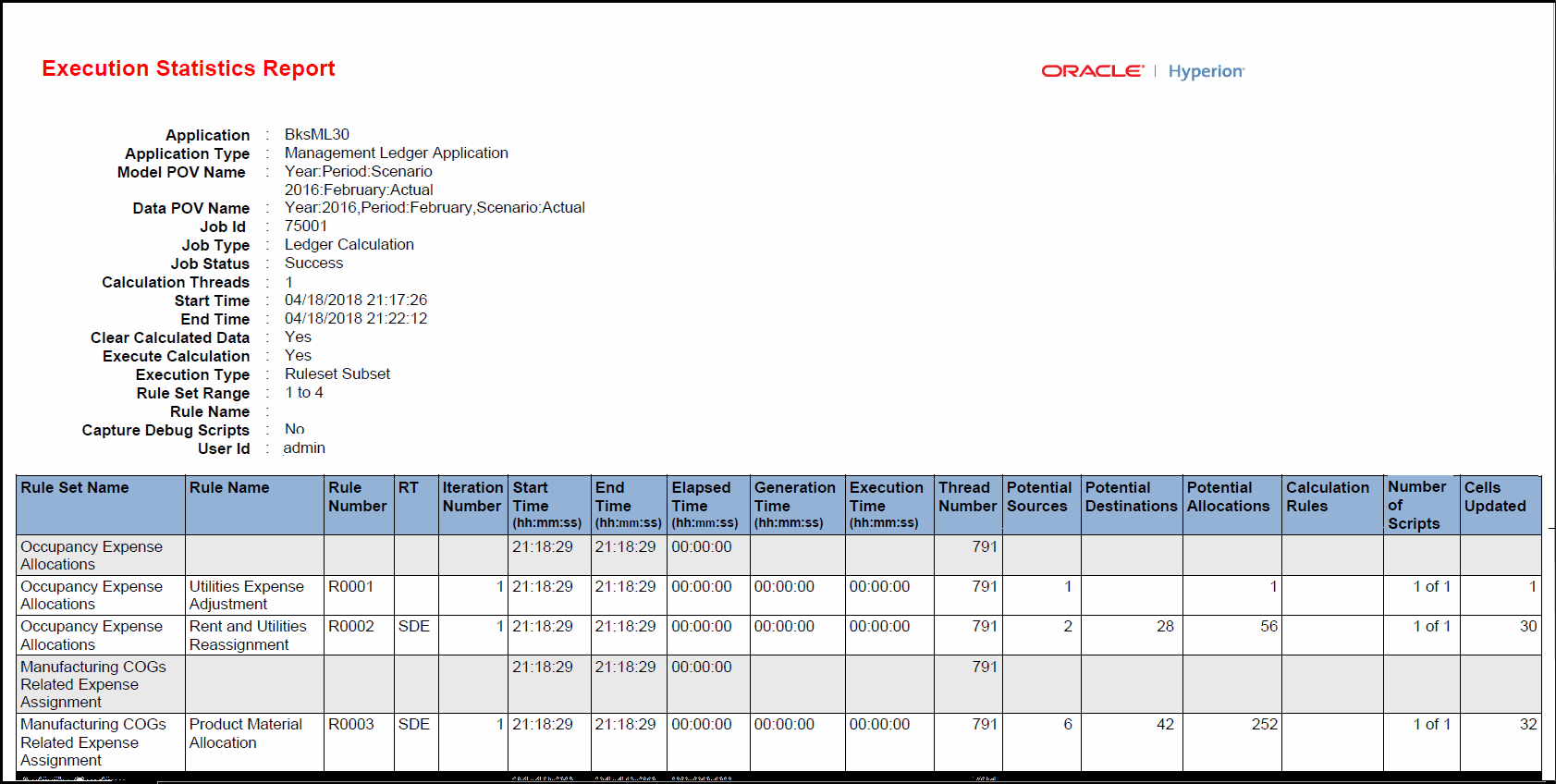## Execution Statistics Report Example

Execution Statistics reports show runtime statistics collected for the selected Ledger Calculation job type following the end of the job.

Figure 13-5 Example of an Execution Statistics ReportThis report shows application name, application type, model and data POV names, job ID, job type, job status, the number of calculation threads, the start and end times, whether calculated data was cleared, whether the calculation ran, the execution type, the rule set range, whether debug scripts were captured, the user ID, rule set names, rule names and numbers, the iteration number, elapsed time, report generation time, thread number, potential sources, potential destinations, potential allocations, the number of calculation subrules that ran compared with the total, the number of scripts that ran compared with the total, and the number of cells updated.

Note:

Calculation Rules, the number of calculation subrules that ran for a rule compared with the total, is only displayed for allocation rules where the destination is the same as the source, but a different dimension is selected. Rules of this type are calculated with a different subrule for each distinct member combination of the matched source dimensions in the source member selection of the rule. For successful calculations, the number of subrules that ran is the same as the total number.